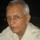1. Home >
2. Apps >
3. Groups >

# An elementary Arithmetic puzzle with a trivial solution

Question asked by Ramani Aswath in #Brainy Puzzles on Mar 5, 2020Ramani Aswath · Mar 5, 2020
Rank A1 - PRO

1+1=2

2+2=11

11+11=?

Posted in: #Brainy PuzzlesRamani Aswath · Mar 7, 2020
Rank A1 - PRO

It is a straight forward addition.

The number system is other than decimal. Really trivial solution. As simple as 1+1=2Ramani Aswath · Mar 9, 2020
Rank A1 - PRO

The most common number systems are: binary, decimal, octal, and hexadecimal. If a number system is based on base 3, it will go like this:

1, 2, 10, 11, 12, 20, 21, 22, 100

So, 1+1=2, 2+2=11

11+11=?Venkat Sai Nakkina · Mar 9, 2020
Rank C3 - EXPERT

In the base 3 system the series will be

1,2,10,11,12,20,21,22,100,101,102,110,111,112,120,121.......

1+1=2

2+2=11

11+11=120Ramani Aswath · Mar 9, 2020
Rank A1 - PRO

11 in ternary is 3^1+1= 3+1=4 in decimal system

So 11+11 in ternary equals 4+4=8 in decimal system, which is 2*3^1+2=22 in ternary.

120 in ternary is 3^2+2*3^1+0=15 in the decimal system.

## You must log-in or sign-up to reply to this post.

Click to Log-In or Sign-Up